# Ohio Graduation Test: Triangles Chapter Exam

Exam Instructions:

Choose your answers to the questions and click 'Next' to see the next set of questions. You can skip questions if you would like and come back to them later with the yellow "Go To First Skipped Question" button. When you have completed the practice exam, a green submit button will appear. Click it to see your results. Good luck!

### Page 1

#### Question 2 2. If angle a is 66 degrees and angle b is 54 degrees, what is the measure of angle d?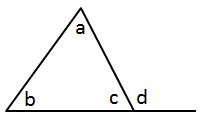#### Question 4 4. In the figure below, Angle 13 = 10y and Angle 11 = 3y + 11. Determine the measure of Angle 16.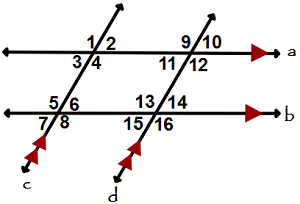#### Question 5 5. What type of triangle is pictured?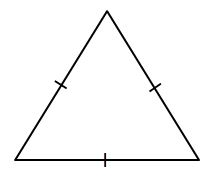### Page 2

#### Question 6 6. Mary claims that the triangles below are similar by AA. Do you agree or disagree with Mary?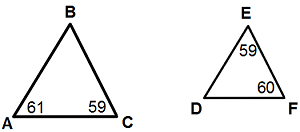#### Question 7 7. What type of triangle is pictured?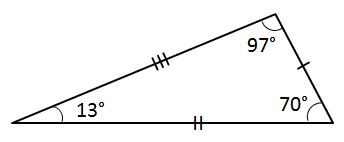#### Question 9 9. What type of line segment is represented by the dotted line in the pictured triangle?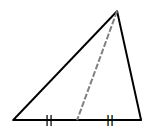#### Question 10 10. What type of triangle is pictured?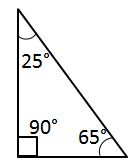### Page 3

#### Question 12 12. In the figure below, Angle 7 = 15x and Angle 10 = 9x + 30. Determine the measure of Angle 10.#### Question 13 13. In the pictured triangle, which of the following lines is an altitude?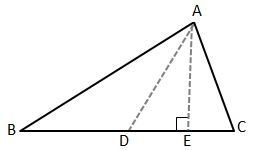#### Question 14 14. Given the triangle PQE, calculate the measure of Angle Q.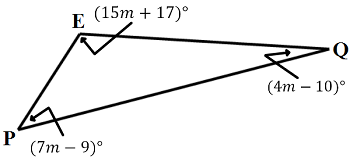### Page 4

#### Question 16 16. Which statement is true regarding areas based on the pictured triangle? Point G is the centroid.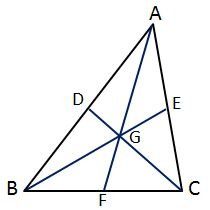#### Question 17 17. Conclude whether triangle BEN is similar to triangle MAT.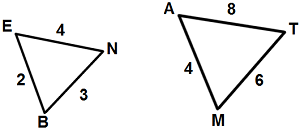#### Question 18 18. In the pictured triangle, what is side A, relative to angle x?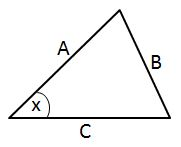#### Question 20 20. If angle a is 60 degrees, what is the measure of angle b?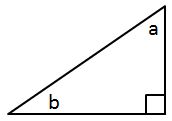### Page 5

#### Question 21 21. Given the triangle ABC, solve for the value of y.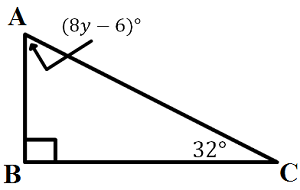#### Question 24 24. In the pictured triangle, what is the centroid?#### Question 25 25. In triangle GBN, Angle G is seven more than three times a number, Angle B is five more than four times the number, and Angle N is twelve less than five times the number. Solve for the measure of Angle G.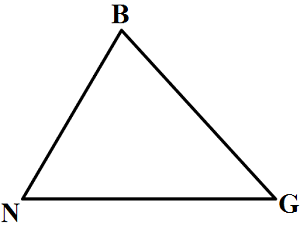### Page 6

#### Question 26 26. What type of triangle is pictured?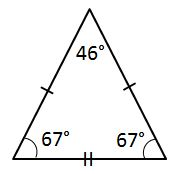#### Question 27 27. In the pictured triangle, what is side B, relative to angle x?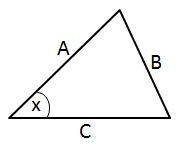#### Question 28 28. If angle a is 80 degrees, what is the measure of angle d?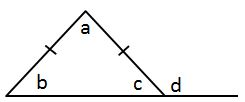#### Question 29 29. What type of line segment is represented by the vertical dotted line in the pictured triangle?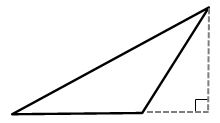#### Question 30 30. Josh claims that these triangles are similar by SSS. Do you agree or disagree with Josh?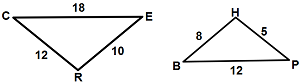#### Ohio Graduation Test: Triangles Chapter Exam Instructions

Choose your answers to the questions and click 'Next' to see the next set of questions. You can skip questions if you would like and come back to them later with the yellow "Go To First Skipped Question" button. When you have completed the practice exam, a green submit button will appear. Click it to see your results. Good luck!

Support